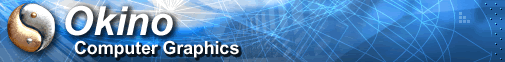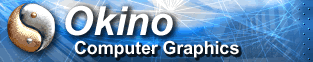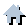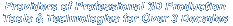You are here:   Home »  Import CAD Formats »  ESRI ShapeFilesESRI Shape (.shp) File Importer

#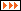Importing ESRI SHP Shape Files

This geometry import converter reads in the point, line and polygon-area geometrical vector data from a ESRI "Shape File". These files are often created using ESRI's ARC/INFO, ARC/VIEW and ARC/GIS. The file extensions are .shp (geometry data) and .cshp (ESRI ShapeFile project file, which makes reference to other .shp files on disk).

If you are provided a .cshp file then load that file only, else load in each .shp file individually.

NOTE: The ESRI shapefile data is often located very far away from the origin, such as 1 million units. For single precision floating point file formats (which is most 3D file formats), these large-unit shapefiles exceed the single floating point precision of 6 digits. This importer defaults the 'Center at origin' option to 'on' so that higher numerical precision can be attained.

##Overview of ESRI ShapeFiles

The ShapeFile format was defined by Environmental Systems Research Institute, Inc. (ESRI) for storing simple vector data elements, most often used for GIS datasets and GIS flat maps.

##Dialog Box Options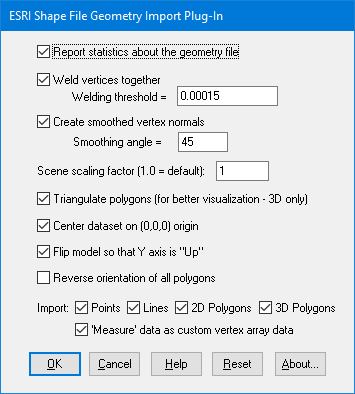These options control how the imported geometry is processed before it is sent to the NuGraf/PolyTrans internal database.

Report Statistics About the Geometry File

If this checkbox is enabled then the import converter will print out the number of objects and polygons that were parsed from the file.

Weld Vertices Together, Threshold =

Polygons read in from a .stl file are, by nature, completely separate from each other and do not share vertices even if two adjacent polygons are situated side by side. This is quite inefficient and also it will not allow the .stl data to be 'smoothed' out using the smoothing option described below. To overcome these problems the 'Welding' option can be enabled (check-marked). Vertex welding collapses adjacent vertices which are within a distance less than or equal to the threshold value specified on the dialog box This welding operation should be performed if the 'Create Smoothed Vertex Normals' option (see below) is enabled. Also, the welding operation can become quite slow for objects with large number of vertices since every vertex must be compared against every other vertex (an n-squared problem). This option is enabled by default.

Threshold Value Type-In

If the distance between two vertices is less than or equal to this number, and the 'Weld Vertices Together' checkbox is check-marked (enabled) then the two vertices will be collapsed (welded) into one. Since no edge in a .stl file should be shorter than 0.00015 units, the minimum value for this threshold value should be set to 0.00015 (which is the default).

Create Smooth Vertex Normals

All .stl files do not contain vertex normals and thus will not look smooth when shaded. This option, when enabled, will allow smoothed vertex normals to be computed for the data based on the angle between adjacent polygons.

The smoothing criterion is based on the angle between adjacent polygons; common smoothed vertex normals will be computed if the angle between their geometric surfaces normals is less than the 'Smoothing Angle' type-in value described below.

If this checkbox is disabled (un-checkmarked) then no new vertex normals will be created.

NOTE: for this option to work properly the input data must have its vertices welded together so that adjacent polygons share the same vertices (the 'Weld Vertices' checkbox must be enabled (check-marked)). This option is enabled by default.

Smoothing Angle Type-in

This type-in value sets the cut-off smoothing angle (in degrees) used by the vertex normal computation algorithm. If the angle between two adjacent polygons is greater than this number then no smoothing will occur (a crease will be evident between the two polygons), else smoothing will occur where the polygons meet. The default angle is 45 degrees; higher values will make the surface smoother.

Scene Scaling Factor (1.0 = default)

This type-in box allows the relative size of the imported dataset to be scaled. Values greater than 1.0 will scale the model larger, while values less than 1.0 will scale the model smaller.

In general terms, you should scale the model so that it fits in a bounding box of 1x1x1 to 10000x10000x10000 units. This will ensure that the best numerical precision is maintained.

Triangulate Polygons

If this checkbox is enabled then all polygons will be triangulated prior to import. ESRI shapefiles often have concave polygons with dozens or hundreds of vertices. Most 3D file formats can only handle polygons with a maximum of 3 or 4 vertices. Also, most DirectX and OpenGL realtime displays will not show the shapefile correctly unless it is triangles or quads. Hence, enable this option if the dataset does not view properly in a downstream 3D program.

Center Dataset at the Origin (0,0,0)

If this checkbox is enabled then the model will be re-centered at the origin. This is the default because many ESRI shapefiles are located very far away from the origin, such as 1 million units away. A single precision floating point number can only represent 6 digits, so vertex coordinates such as 1000000.12345 will get truncated to 1000000.0 due to numerical limits. By centering the dataset at the origin the numerical precision is maintained to a higher degree.

Flip Model so that Y Axis is 'Up'

If this checkbox is enabled then the model will be reorientated so that the 'Up' axis of the model will be aligned with the positive Y axis (which is used by the converter) instead of the positive 'Z' axis as used by .stl files. This option should be enabled by default.

Reverse Orientation of All Polygons

If this checkbox is enabled then the orientation of each polygon will be reversed so that the polygons' normals point in the opposite direction. By default this option is disabled.

Import:

These checkboxes allow specific entities from the ESRI shape file to be imported selectively.

Points

Enabling this checkbox will allow 2D and 3D points to be imported.

Lines

Enabling this checkbox will allow 2D and 3D line segments to be imported.

2D Polygons

Enabling this checkbox will allow 2D polygons to be imported. These polygons only have XY coordinates.

3D Polygons

Enabling this checkbox will allow 2D and 3D points to be imported. These polygons have XYZ coordinates.

'Measure' data as custom vertex array data

Enabling this checkbox will allow "Measure" data to be imported from the file. This is extra numeric data often associated with points inside a ESRI Shape file.